Manfred MOHRDimensions I (1978-1980)

This work phase (1978-79) is based on the four-dimensional hyper-cube. In this work the graph of a hyper-cube is the basic generator of signs. This graph is a two-dimensional representation of the hyper- cube, indicating relationships between points, lines, squares and cubes, inherent in this structure. A global structure is generated either by combinatorially selecting lines from the cube or by showing connecting paths between given points on the graph (diagonals).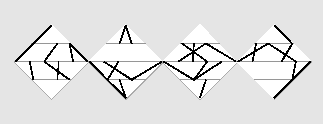P-224/A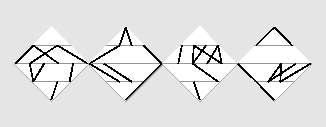P-224/B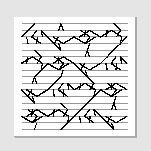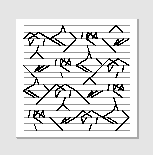P-226/A P-226/B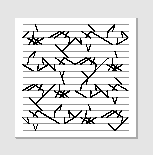P-226/C